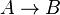# Number of explainers and learners

Jump to: navigation, search

This page classifies learning situations by the number of explainers (e.g. teachers) and number of learners (e.g. students) present. Each learning situation can be visualized as a directed graph where an arrow from$A$ to$B$ ($A \to B$) means "$A$ explains to$B$".

Number of explainers Number of learners Condition on identity of explainers and learners Examples
one one the explainer is not the learner one-on-one tutoring
one one explainer is the learner self-studying, solitary original research
one many the explainer is not one of the learners classroom learning, textbook, blog post explaining an idea, one tutor with many students
one many explainer is one of the learners a group of students studying together and one of them is explaining something, a student-teacher who teaches a class and is simultaneously learning how to teach
many one the learner is not an explainer parents explaining something to a child
many one the learner is also an explainer but there are other explainers who are not the learner an autodidact who also receives guidance from a tutor nearby
many many none of the explainers are a learner classroom with multiple teachers, textbook with multiple authors
many many each explainer is also a learner a mutual exchange of information in a conversation, collaborative original research
many many some of the explainers are not learners, some of the explainers are learners a classroom with one teacher and one-student-teacher who take turns teaching